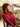# Lets code the TDD way!

Posted on September 16, 2018 - 5 min read

TDD — Test Driven Development.

Testing is a crucial part of software development. It is very important for finding bugs, verifying the functionality and improving the general stability of any piece of software. Almost all developers test their code in one way or the other. There are basically two ways to test:

• Manually run the program and try out all functionality to see if everything is working as expected.
• Write automated tests that are or will run every time the code changes, (this is probably the best way).

And because '2' is the best way we will be focusing on that, but first let's understand what exactly TDD is

What is Test Driven Development?

TDD is a software development approach where the test is written out or defined before actual development is done. It actually follows a defined pattern which goes as follows:

• Write some tests
• Run all tests with the expectation that they will all fail
• Write some code
• Run tests
• Refactor code until all the tests pass

And this method is often referred to as RED-GREEN-REFACTOR

There are a few advantages that come with adopting this pattern of software development

• cleaner and more concise code
• A deeper understanding of the functionality of the software
• Good test leads to lesser bugs during the production or deployment of a software

A practical example of TDD using Javascript

This practical example will mainly focus on an introduction to mocha, chai and environment setup for writing tests in ES6.

Mocha - This is a BDD testing framework, that runs both on Nodejs and in the browser. It has so many little pieces to it but there are just a few that are needed for our test.

• describe()

Your test suites may consist of many parts and the describe block can be used to distinguish between them in a clear manner. It takes in 2 parameters - the description and the function that will contain your test afterward.

``````describe("Function A", () => {
// Test for function A here
})
describe("Function B", () => {
// Test for function B here
})``````

• it()

This is where your code for the test will be located

``````describe("Function A", () => {
it("should equal 9", () => {
expect(FunctionA(6, 4)).to.be.equal(9)
})
})``````

and if you are writing an asynchronous test - which means that your testing code may end later without the whole block waiting for it, you can pass a done to the callback function of it()

``````describe("Function A", () => {
it("should return 2 when we pass 1 and 1", function(done) {
done()
})
})``````

• hooks

Because of its BDD style, Mocha provides hooks ( before(), after(), beforeEach(), and afterEach()) that can be used to set up preconditions and clean up after your tests.

``````describe("Function A", () => {
before(() => {
// runs before all tests in this block
})
after(() => {
// runs after all tests in this block
})
beforeEach(() => {
// runs before each test in this block
})
afterEach(() => {
// runs after each test in this block
})
it("should do something", () => {
// test codes here
})
})``````

Chai - This is a BDD / TDD assertion library for Node and the browser that can be delightfully paired with any javascript testing framework. It majorly provides 3 types of assertions which can all be found here

https://www.chaijs.com/guide/styles/

Setup for ES6 Testing:

We are going to write a function to get the factorial of a number and since we are going by the TDD way we would go ahead and write the test for our function. First, we have to install our needed dependencies - Mocha and Chai

``````npm init --yes
npm i mocha chai -D``````

Let's also go ahead and setup babel for converting our code into a compatible version by installing the following

``npm i babel-register babel-preset-env babel-preset-stage-0 -D``

Then, create a `.babelrc` file and add the following

``````{
"presets": [
"env",
"stage-0"
]
}``````

``````//import libraries needed for assertion
import { assert } from "chai"

//import the main file for test
import computeFactorial from "../src/main.js"

describe("factorial", () => {
describe("handle valid input", () => {
it("should return 6 as factorial for 3", () => {
assert.equal(computeFactorial(3), 6)
})
it("should return 120 as factorial for 5", () => {
assert.equal(computeFactorial(5), 120)
})
})
describe("handle invalid input", () => {
it("should return undefined as factorial for -5", () => {
assert.equal(computeFactorial(-5), "undefined")
})
it("should return undefined as factorial for 'demo'", () => {
assert.equal(computeFactorial("demo"), "undefined")
})
})
})``````

Without adding our factorial function we can go ahead and run this test expecting that it would fail because the factorial function is not present

``````const computeFactorial = value => {
if (typeof value != "number") {
return "undefined"
} else if (value < 0) {
return "undefined"
} else {
let factorial = 1
for (let i = value; i >= 1; i--) {
factorial = factorial * i
}
return factorial
}
}

export default computeFactorial``````

To run our test lets go ahead and edit the `scripts` line in our `package.json`

``````"scripts": {
"test": "mocha test/*.js --require babel-register --reporter spec -exit"
}``````

Then run the test with the command `npm test` and you'll get the following result

I hope you enjoyed reading this article, please reach out to me if you have any feedback or ways I can improve things.